All submissions of the EM system will be redirected to Online Manuscript Submission System. Authors are requested to submit articles directly to Online Manuscript Submission System of respective journal.

Original Article
, Volume: 6( 1)

# The Possibility of FTL Space Travel by using the Quantum Tunneling Effect through the Light Barrier

*Correspondence:
Musha T, Advanced Sci-Tech Research Organization, 3-11-7-601, Namiki, Kanazawa-Ku, Yokohama 236005, Japan, Tel: +81457857426; E-mail: [email protected]

Received: April 03, 2017; Accepted: May 10, 2017; Published: July 07, 2016

Citation: Musha T. The Possibility of FTL Space Travel by using the Quantum Tunneling Effect through the Light Barrier. J Space Explor. 2017;6(1):121.

### Abstract

In normal physics, nothing can move faster than the speed of light, because Einstein's theory of relativity forbids it. However, some physicists have proposed a model of faster-than-light (FTL) travel, formulated in the context of Lorentzian manifolds, which are used in general relativity to construct space-time models, called a wormhole and an Alcubierre’s warp drive. Warp drive works by distorting the fabric of the spacetime, contracting spacetime in front of the spaceship and expanding it behind to create a warp continuum bubble between the two spacetime distortions in front of and behind the craft. However, it has been shown that the total integrated energy density needed to maintain the warp metric is physically unattainable. In this article, the author presents another possibility of a FTL travel method which applies the tunneling effect through the light barrier caused by the manipulation of ZPF field surrounding the spaceship.

### Keywords

Warp drive; Tunneling effect; FTL travel; Tachyon; ZPF field

### Introduction

Some physicists have proposed a model of faster-than-light (FTL) travel, formulated in the context of Lorentzian manifolds, which are used in general relativity to construct space-time continuum models . In normal , nothing can move faster than the speed of light. Einstein's theory of relativity forbids it. There are two exceptions to this rule however. The first is what's commonly called a worm hole, a bridge connecting two different parts of space . A ship crossing this bridge would move at below light speed, but still arrive before a beam of light that would have had to go the long way around. Warp drives are the second and more appealing option. A ship can't move through space faster than the speed of light. But with enough energy, space itself can move faster than the speed of light. The most renowned theory is the Alcubierre drive, which is possible to circumvent the problem by suitably warping space-time . The Mexican physicist Mique Alcubierre originally developed the idea in the 1990's, named an Alcubierre warp drive which would create a bubble of energy behind the ship and a lack of energy in front of the ship, like a giant cosmic wave a space ship could surf. That particular section of space can travel faster than the speed of light in the surrounding space, and anything on or in that bubble will accelerate with it. Finazzi et al. propose creating this bubble of space-time by using a massive amount of "exotic matter," or dark energy , but how this bubble would be created is still a mystery and they give no knowledge as to how a warp bubble might actually be established. According to their calculations, it would take a huge amount of energy to create the bubble, and then increasing amounts of energy to contain the highly repulsive dark energy. Another method is based on general relativity which permits the travel of an object at a greater velocity than that of light by bending the space-time itself. If space-time is warped properly, the velocity of traveling object does not exceed the speed of light, but it appears to be moving faster than light to observers in normal space-time. If certain quantum inequalities conjectures by Ford and Roman hold, it was shown by Pfenning and Ford  that the energy requirement for some warp drives became the energy equivalent of 1064 kg to transport a small spaceship across our galaxy. The author proposed in his paper that there is a possibility of light particles which can penetrate through the light barrier by the violent acceleration , which suggests that electron neutrinos travels in a space in a FTL mode as confirmed many experiments. Recently, a group of physicists have been working to measure the neutrinos generated from a particle accelerator at CERN. This group discovered neutrinos arriving faster than would have been expected and they appear to be traveling faster than the speed of light itself, but they draw no definitive conclusions [7,8].

In this article, the author presents an advanced theory that the spaceship can be traveled at FTL speed by applying the theory of tunneling effect through the light barrier.

Possibility of Tunneling Through the Light Barrier

From the wave function for the particle given by(1)

the wave equation for an accelerating particle can be described as(2)

where ψ is a wave function of the particle,is a Plank constant divided by 2π , p is its momentum, c is a light speed, H is a Hamiltonian given by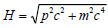, and a is a proper acceleration given by p= m0 at , where m0 is a proper mass.

From Eq. (2), we have the solution given by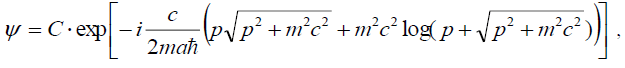(3)

where C is an arbitrary constant.

From which, we obtain

(subluminal speed: v < c )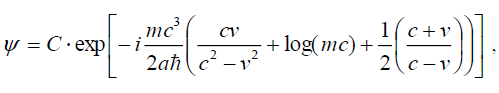(4)

(superluminal speed: v* > c )(5)

where m* is an absolute value of the imaginary mass and v* is a velocity of the superluminal particle. Figure. 1. shows the wave function for the highly-accelerated particle, which shows the possibility of tunneling through the light barrier.

We consider the light barrier as a potential barrier, the probability of a particle to tunnel through the light barrier can be estimated according to the WKB approximation shown as .(6)

where β*=v* / c and T is a probability to become FTL state

Supposing that m = m* , the probability of a spaceship composed of N elementary particles to plunge into a FTL state can be roughly estimated as follows when we let β*→∞:(7)

Thus it can be seen that violent acceleration makes possible for the spaceship to tunnel through the light barrier as shown in Figure. 2.

However, it can be estimated from Eq. (7) that enormous acceleration over 1060 ( m/ sec )2 m is required for the spaceship with the proper mass of 100 tons to plunge into a FTL state, which cannot be attained by the conventional propulsion systems.

Acceleration generated by the manipulation of the cut-off frequency of ZPF spectrum

By the Puthoff’s theory on zero-point-fluctuation (ZPF) field , the rest mass of the pion corresponding to ZPF field can be given by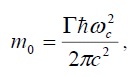(8)

whererepresents a damping constant for Zitterbewegung oscillations.

As the force acting on the mass of the body can be described by, then we have. Under Under the condition that f = 0, we have. then enormous acceleration can be produced when the cutoff frequency of ZPF spectrum is reduced to be zero around the spaceship from Eq. (8) shown as follows;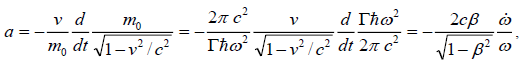(o)

where ω an upper frequency of ZPF spectrum and β=v / c .

From Eqs. (7) and (9), the probability to tunnel through the light barrier is given by(10)

When we let, where, then we have. By inserting them into Eq. (10), the probability to tunnel the light barrier of the spaceship becomes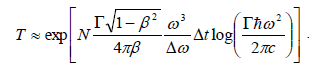(11)

Supposing that β=0.1, Δω=ωc and Δt =1.0 in Eq. (11), the tunneling probability of the spaceship with the mass of 100 tons, that can plunge into FTL state versus the cutoff frequency of ZPF field can be shown in Figure. 3. From which, it is possible to travel at the FTL state if we can decrease the mass of the spacecraft by the manipulation of ZPF field around the space ship.

Possibility to manipulate ZPF field around the spaceship

If the wave energy emitted by a spaceship in the form of beam interacts with vacuum electromagnetic zero-point field (ZPF), it perturbs ZPF and decreases zero-point radiation pressure, the zero-point radiation pressure in the rear vicinity of spaceship acting upon spaceship. For decreasing ZPF energy, we can utilize negative energy radiation. There are two main approaches to generate negative energy, which are the Casimir effect and the squeezed vacuum. The second way to obtain negative energy is to squeeze quantum vacuum by ultrahigh intensity lasers. By reducing the energy below the zero-point fluctuation of vacuum, the vacuum becomes squeezed, resulting in negative energy. Davis and Puthoff  proposed a method by use of an ultrahigh intensity laser coupled with fast moving mirrors. By their method, negative energy can be generated by an array of ultra-intensity lasers using an ultra-fast rotating mirror system to create the squeezed state of the electromagnetic field, both pulses (positive and negative) are equal in time interval release. Rapidly rotating mirrors in this setup would serve the purpose of separating the positive and negative energy, if the beams hit the mirror at a very shallow angle.

Another method would be to superimpose photons upon each other in order to create a beam of negative energy proposed by Pfenning , the way to squeeze light would be to manufacture extremely light containing train of pulses and combine them together to create squeezed state. For the squeezed vacuum state, the density is given by(12)

where L3 is the volume of a large box with the length L and r is the squeeze parameter related to the expectation of photon numbers.

By utilizing these methods, negative energy have just become available.

This suggests that one might conceivably surround a spaceship with a “bubble” of highly energy-depleted (negative energy) vacuum, which makes possible to decrease the mass of the spacecraft to induce violent acceleration, which makes possible to jump into a FTL mode by quantum tunneling effect as shown in Figure. 4.

FTL travel of the spaceship in a tachyonic state

From the bosonic string world-sheet action given by(13)

the spectrum of bosonic strings contains a tachyonic mode for open strings  shown as(14)

which allows the existence of negative energy, that leads to the instability of the vacuum. This means that the tachyon is an unstable particle, which is allowed to appear for a short period of time in empty space and the FTL state of a spaceship maintained within a short period of time. From the uncertainty relation for the tachyon shown as follows , we can estimate the distance of the spaceship in a FTL state;(15)

From which, the difference of momentum before and after penetrating light barrier is(16)

If v*→∞, then, thus Eq. (16) becomes(17)

when we suppose m*=m0 .

From Eq. (15), the traveling distance of the space ship in a FTL state becomes(18)

In the case when β<<1 and v0 / v* is negligibly small, the traveling distance of FTL travel can be roughly estimated by(19)

from Eq. (8), which calculation result is shown in Figure. 5.

Figure 5: Traveling distance of the spaceship in a FTL state vs. ZPF frequency around the spaceship.

From Figure. 3. the radial frequency to plunge into FTL state for the spaceship with the mass of 100 tons can be estimated to be about 104 Hz, then the spaceship can travel in a FTL state with a distance of 1.5 × 1024 m from Eq. (19), which is beyond the distance to the near-by galaxies. Thus, it is shown that tunneling FTL travel can be possible if negative energy can be utilized which is also used for the construction of worm holes . The total negative energy required to cancel the positive ZPF field surrounding the spaceship can be roughly estimated by(20)

if we let R =50m,which is a radius of the negative energy surrounding the space ship, ωc=1.2 ×1024 /s and

ω0=104/s.

As the equivalent mass of which becomes 4.6 × 1024 kg, then it is required about a half of the Earth’s mass to transport a small spaceship between galaxies. However,ωc is much lower value, we don’t need so much negative energy.

Thus, we suppose that there is some sort of quantum tunneling that might allow a subliminal particle to become a superluminal particle, it is considered that we could tunnel through such a light barrier using quantum effect to attain superluminal velocities for space travel.

### Conclusion

In this paper, the author presents an advanced theory that the spaceship can be traveled at superluminal speed by applying the Puthoff’s theory on ZPF field. From the theoretical analysis, it can be seen that the FTL travel can be achieved by the quantum tunneling effect induced by the manipulation of the ZPF spectrum surrounding the spaceship.

### References

Select your language of interest to view the total content in your interested language

###### Citations : 214

Journal of Space Exploration received 214 citations as per Google Scholar report

#### Indexed In

• Index Copernicus
• Open J Gate
• China National Knowledge Infrastructure (CNKI)
• Cosmos IF
• Directory of Research Journal Indexing (DRJI)
• Secret Search Engine Labs